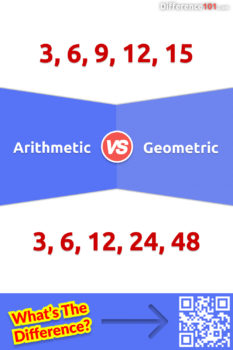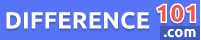# Arithmetic vs. Geometric: What is the Difference Between Arithmetic and Geometric?

Arithmetic and geometric sequences are two types of sequences. In this article, we'll explain the difference between these two types of sequences, and give examples of each.Both arithmetic and geometric are sequences of numbers but have distinct characteristics. The biggest difference between arithmetic vs geometric series lies in the type of difference between the consecutive terms. The consecutive terms of an arithmetic sequence have a constant difference while the terms of a geometric difference are in a constant ratio.

Let’s take a closer look at arithmetic vs. geometric here:

## What is Arithmetic?

An arithmetic sequence is the one in which each successive term differs from the previous one with a constant difference.

## What is Geometric?

A geometric sequence is the one in which each successive term differs from the previous one with a constant ratio.

## Arithmetic Pros and Cons

### Pros of Arithmetic

• It is easy to calculate.
• It is finite since it follows a fixed pattern.

### Cons of Arithmetic

• It cannot accurately average ratios and percentages.
• It cannot be used for averaging highly skewed data.

## Geometric Pros and Cons

### Pros of Geometric

• It is defined rigidly and follows a pattern.
• One can undertake further algebraic treatment through a geometric sequence.

### Cons of Geometric

• It is difficult to calculate.
• Understanding a geometric pattern can be quite tough at times.

## Arithmetic vs Geometric Sequence Examples

### Examples of Arithmetic

• The sequence 1, 4, 7, 10, 13, 16 is an arithmetic sequence with a difference of 3 in its successive terms.
• The sequence 28, 23, 18, 13, 8 is an arithmetic sequence with a difference of 5 in its successive terms.

### Examples of Geometric

• Sequence 2, 6, 18, 54, 162 is geometric sequence where each successive term is 3 times the previous one.
• The sequence 64, 16, 4, 1 is a geometric sequence where each successive term is one-fourth of the previous one.

## Bringing out the Similarities between Arithmetic and Geometric for Better Understanding

• Both arithmetic and geometric are sequences.
• Both these sequences follow a pattern.
• Both these sequences can be divergent.
👉  AWD vs. 4WD: What's the difference between AWD and 4WD?

## Arithmetic and Geometric FAQs

### What are Arithmetic and Geometric Sequences?

An arithmetic sequence is one where the next value is obtained by adding or subtracting a constant to its preceding value. To obtain a geometric sequence, the next value in a sequence is arrived at by dividing or multiplying a constant to the preceding term.

### How are Arithmetic and Geometric Sequences Similar?

Both arithmetic and geometric sequences have a constant difference between their two consecutive values and this pattern can not be broken in the sequence.

### What is the Difference Between Arithmetic and Geometric Progression?

An arithmetic progression series has a constant value that is either added to or subtracted from the previous term. Whereas a geometric progression series has a constant value that is either multiplied or divided by the previous term. Arithmetic vs geometric return also differs based on these two progressions.

👉  DOC vs. DOCX: What Is the Difference Between DOC and DOCX?

### What makes an Arithmetic Sequence?

A definite increasing or decreasing pattern makes an arithmetic sequence.

### What are the Examples of Arithmetic Sequences?

A few examples of an arithmetic sequence are pyramid structures, the seating arrangement in a multiplex-theater or stadium, or temperature changes with changes in sea levels.

### What is the Difference Between Geometry and Mathematics?

Geometry is a subset or a branch of a set called mathematics. Where geometry deals just with establishing relationships between various shapes, mathematics deals with establishing relationships between numbers.

### What is the General Rule of Geometric Sequence?

The general rule of a geometric sequence is to multiply or divide a term by a non-zero constant to get the next term. The sequence is in the form of a, ar, ar², ar³, and so on.

### What is the Difference Between Arithmetic Series and Geometric Series?

An arithmetic series has a constant difference between two consecutive terms. Whereas a geometric series has a constant ratio between two consecutive terms. Arithmetic vs geometric mean also differ based on the difference in these two series.

### What are the Advantages of a Geometric mean?

Following are a few advantages of a geometric mean:

• Any fluctuations in the samples do not affect the sequence much.
• It gives enough weightage to the small sample values against the larger ones.
• It is best suited to find out the relative changes.
• It can be used for further mathematical treatments like algebra.

### How is Arithmetic Used in Real Life?

Arithmetic is used in real life in various ways like making budgets, deciding salary hikes, cooking recipes, exercising or playing games, increasing or decreasing wave generation, determining returns on investment, et al.

### What is the Difference Between Arithmetic Average and Geometric Average?

The major difference between arithmetic vs. geometric average lies in the consideration of compound values. Arithmetic averages do not consider the effect of compounding while geometric averages do consider it. Arithmetic vs geometric growth also differs because of this compounding.

## Conclusion

Both arithmetic and geometric are numerical sequences following a fixed pattern and can be determined. But they differ in how the sequence is obtained. An arithmetic series has a constant difference between its two consecutive terms. On the other hand, the consecutive terms of a geometric series differ by a constant ratio.

#### Alex Stantor

Alex Stantor is a Sorbonne University (Paris, France) graduate in Philosophy and Data Analysis. Currently, he is an Author and Researcher at Difference 101, he writes articles/blog posts on topics such as "thinking differently" and "the importance of difference". Alex is a passionate advocate of diversity in the workplace and in companies, and diversity and inclusion in corporate communications. He currently lives in Brooklyn.

We will be happy to hear your thoughts